Author: Oscar Cronquist Article last updated on May 17, 2022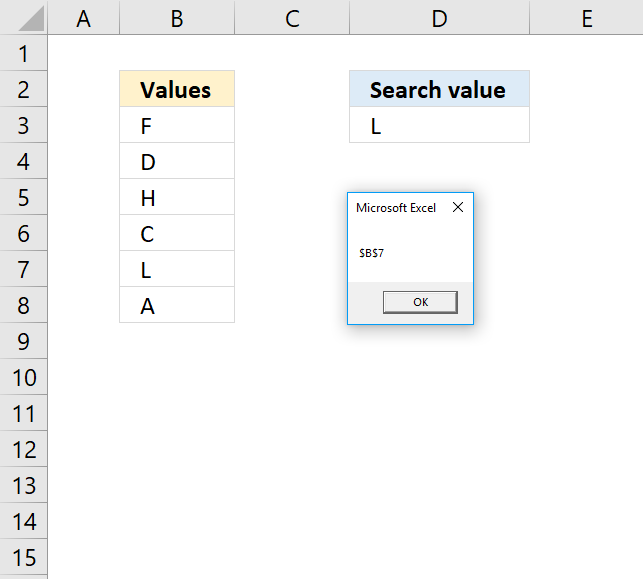The Range.Find method returns a range object for the first cell that matches the lookup value.

Macro used in workbook above

```Sub Macro1()

End Sub
```

The macro above uses the Range.Find method to search cell range B3:B8 for the lookup value in cell D3. A message box shows the address of the found cell.

## 1. Range.Find Syntax

expression.Find( What , After , LookIn , LookAt , SearchOrder, SearchDirection , MatchCase , MatchByte , SearchFormat )

## 2. Range.find Arguments

 expression Required. Range object. what Required. The lookup value. after Optional. Where you want the search to begin. Note, it begins searching the cell after this cell. Must be a single cell. Default cell is A1. lookin Optional. XlFindLookIn constants: xlFormulas - xlValues - xlNotes - lookat Optional. XlLookat constants: xlWhole xlPart searchorder Optional. XlSearchOrder constants: xlByRows xlByColumns searchdirection Optional. XlSearchDirection constants: xlNext (1) - Search for the next matching value in range. xlPrevious (2) - Search for the previous matching value in range. matchcase Optional. True - Case sensitive search. Default value is False. matchbyte Optional. For double-byte characters support. True - Double-byte characters match double-byte characters. False - Double-byte characters match single-byte characters. Default value. searchformat Optional.

The Find method is not going to change the selection or the active cell, it only returns the range object of the found value.

The LookIn, LookAt, SearchOrder, and MatchByte are automatically saved each time you use the Find method. The saved values are used if don't specify these arguments. The Find dialog box changes these settings as well and vice versa meaning the Find method changes the Find dialog box settings. Make sure you specify these arguments each time you use the Find method to prevent unpredictable behavior.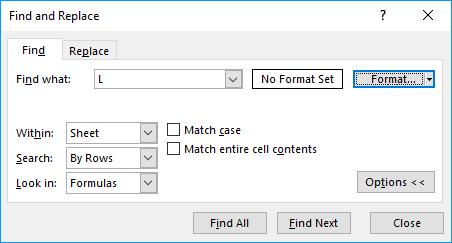It also looks like that the what argument is also saved to the Find dialog box.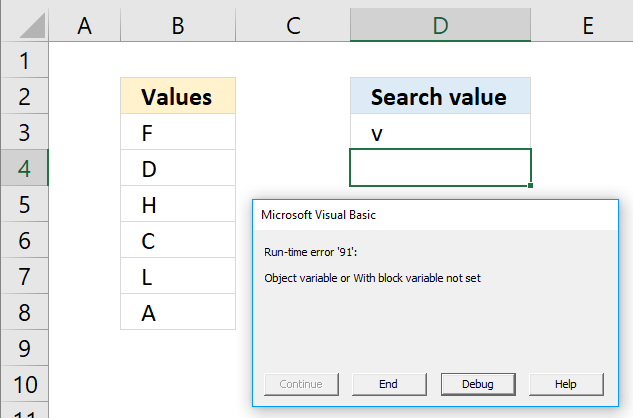The macro returns an error if the value is not found. Run-Time error '91': Object variable or With block variable not set. To handle the error see the following macro.

```Sub Macro2()

If Range("B3:B8").Find(Range("D3")) Is Nothing Then
Else
End If

End Sub
```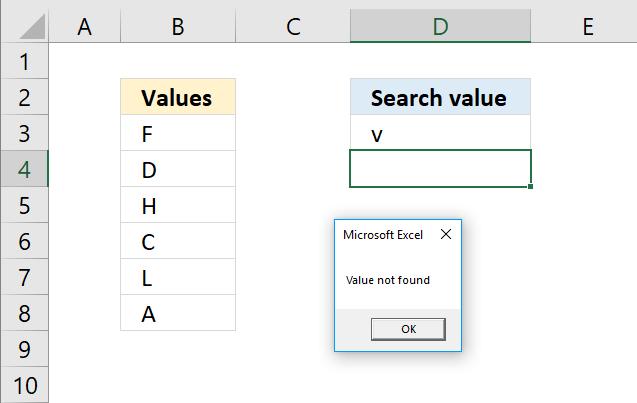## 5. Example 2 - Find multiple values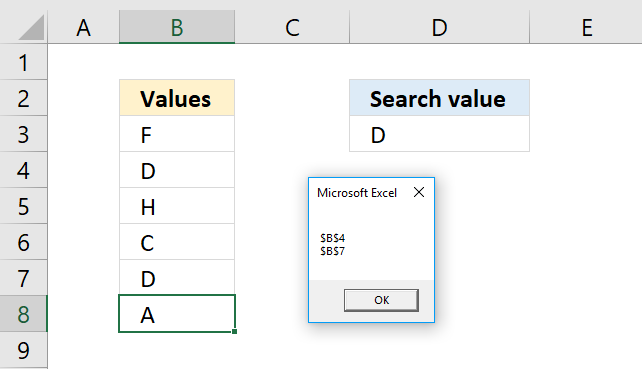The following macro iterates through each found value and saves the cell address to a variable, the msgbox then shows the variable.

```Sub Macro3()
With Range("B3:B8")
If .Find(Range("D3")) Is Nothing Then
Else
Set a = .Find(Range("D3"))
Set b = a
c = c & vbNewLine & .FindNext(b).Address
Set b = .FindNext(b)
Loop
End If
End With
MsgBox c
End Sub
```

## 6. Example 3 - Return adjacent value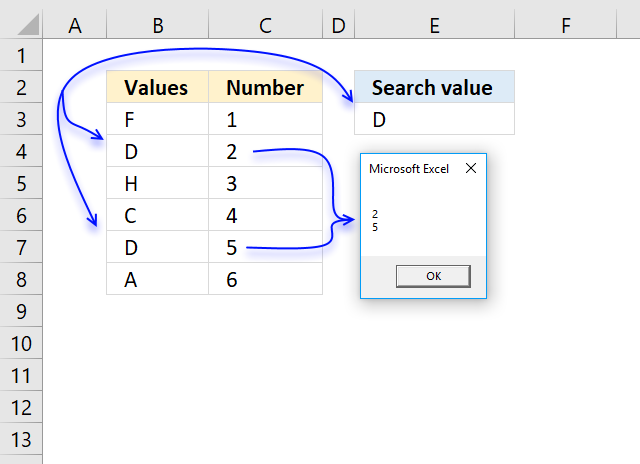The image above demonstrates the macro below, it saves the adjacent values to each found value in cell range B3:B8 using the lookup value in cell E3 to a variable. The msgbox then shows the contents of the variable.

```Sub Macro4()
With Range("B3:B8")
If .Find(Range("E3")) Is Nothing Then
Else
Set a = .Find(Range("E3"))
Set b = a
c = a.Offset(, 1).Value
c = c & vbNewLine & .FindNext(b).Offset(, 1).Value
Set b = .FindNext(b)
Loop
End If
End With
MsgBox c
End Sub
```